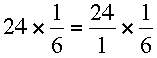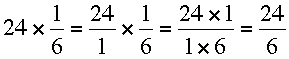SEARCH HOMEMath Central Quandaries & QueriesQuestion from Laurie, a student:         1 24 x -         6 I don't no where the 24 goes and if the number must be the same to divide into the numerator and the denominator . Thankyou for your time.Hi Laurie,

You can think of 24 as 24/1 soand to multiply two fractions you multiply the numerators and denominators soNow you can divide 6 into 24 and get 4.

PennyMath Central is supported by the University of Regina and The Pacific Institute for the Mathematical Sciences.